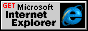# left Property

Internet Development Index

Sets or retrieves the left coordinate of the rectangle surrounding the object content.

Syntax

`TextRectangle.left [ = iCoord ]`

Possible Values

 iCoord Integer that specifies or receives the left coordinate of the rectangle, in pixels.

The property is read/write. The property has no default value.

Expressions can be used in place of the preceding value(s), as of Microsoft® Internet Explorer 5. For more information, see About Dynamic Properties.

Remarks

To access the left coordinate of the second text rectangle of a TextRange object, use this syntax:

```oRct = oTextRange.getClientRects();
oRct.left;```

Note that because the collection index starts at 0, the second item index is 1.

To access the left coordinate of the bounding rectangle of an element object, use this syntax:

```oBndRct = oElement.getBoundingClientRect();
oBndRct.left;```

Example

This example uses the getBoundingClientRect method to retrieve the coordinates of the bounds of the text rectangles within the element.

```<SCRIPT>
function getCoords(oObject) {
oBndRct=oObject.getBoundingClientRect();
alert("Bounding rectangle = \nUpperleft coordinates: "
+ oBndRct.left + " " + oBndRct.top +
"\nLowerright coordinates: "
+ oBndRct.right + " " + oBndRct.bottom);
}
</SCRIPT>
<BODY>
<P ID=oPara onclick="getCoords(this)">```